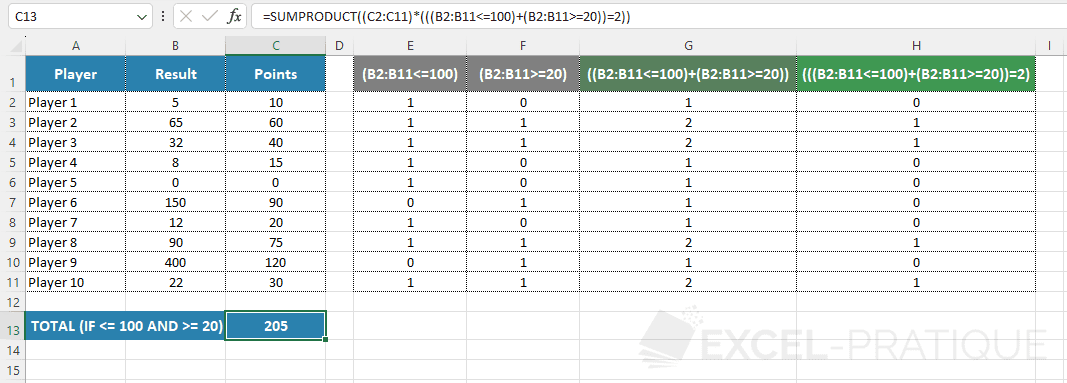# Excel Function: SUMPRODUCT

The SUMPRODUCT function returns the sum of ranges of values multiplied by each other, row by row.

Usage:

`=SUMPRODUCT(range_1)`

or

`=SUMPRODUCT(range_1, range_2, ...)`

## SUMPRODUCT usage example

To better understand the operation of SUMPRODUCT and what its definition of "sum of products" means, here is a simple example to start with: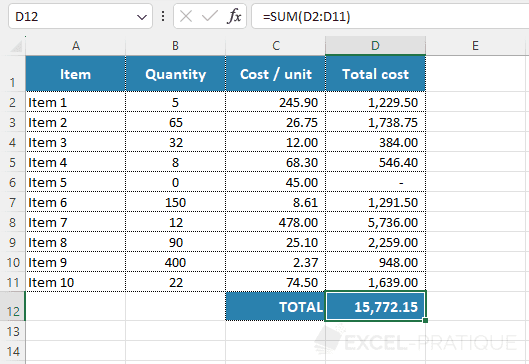In this first table, the total costs in column D are obtained by multiplying the values in columns B and C using the formula `=B2*C2` and the total is simply the sum of these different products `=SUM(D2:D11)`.

The goal now is to calculate the sum of these different products directly (without a "Total cost" column):To do this, insert the SUMPRODUCT function and then add the 2 ranges of cells containing the data to be multiplied by each other (row by row) and for which you calculate the sum: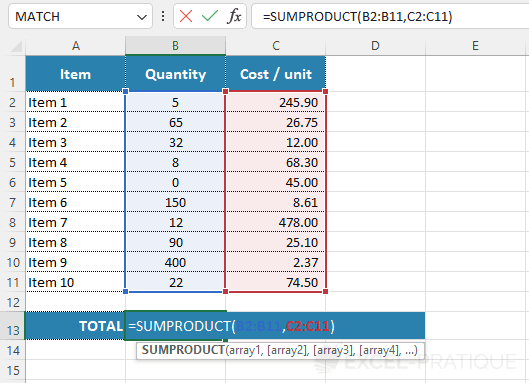The SUMPRODUCT function indeed returns the same total as in the first example:

``=SUMPRODUCT(B2:B11,C2:C11)``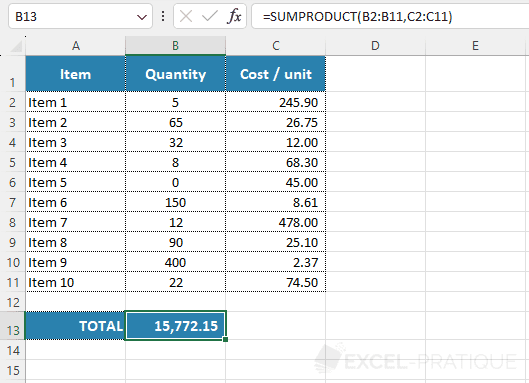Note that the same result can be obtained with the formula:

``=SUMPRODUCT((B2:B11)*(C2:C11))``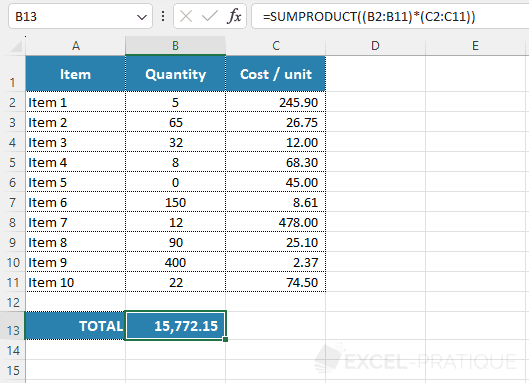In this case, the product of the 2 ranges `(B2:B11)*(C2:C11)` is calculated and returned as a single range for which the SUMPRODUCT function returns the sum.

## SUMPRODUCT with a condition

To perform the same calculation but only for items with a quantity greater than 100, first copy the last formula and add the condition `>100` to it.

The formula then becomes:

``=SUMPRODUCT((B2:B11>100)*(C2:C11))``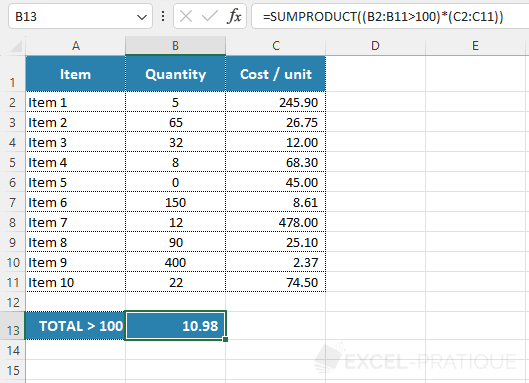This formula does not return the expected result because `(B2:B11>100)` does not contain any quantity, it is instead a range that returns 1 (TRUE) or 0 (FALSE) depending on the `>100` test.

It is equivalent to the previous SUMPRODUCT function (without condition) with these values: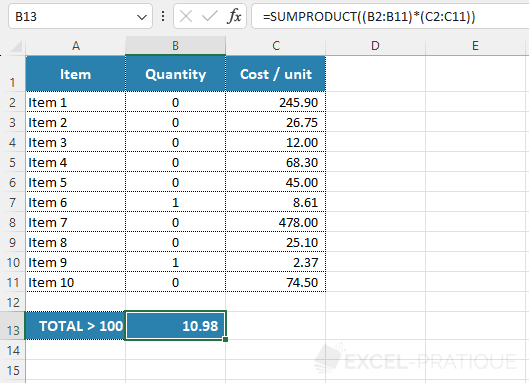In this case, you therefore need to add the condition in addition to the other 2 ranges:

``=SUMPRODUCT((B2:B11)*(C2:C11)*(B2:B11>100))``

Here, the quantity is multiplied by the cost per unit and then by 0 or 1 depending on the test (the products that should not be taken into account will be multiplied by 0 and therefore will not modify the sum).

This time, the SUMPRODUCT function correctly returned the sum of the products taking into account the condition: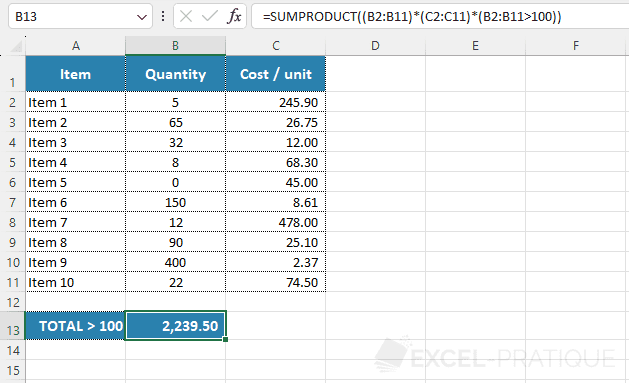## SUMPRODUCT with multiple conditions

To simplify the formula a bit (before complicating it), the SUMPRODUCT function will here need to return the sum of the players' points with a result `>100`.

The formula then is:

``=SUMPRODUCT((C2:C11)*(B2:B11>100))``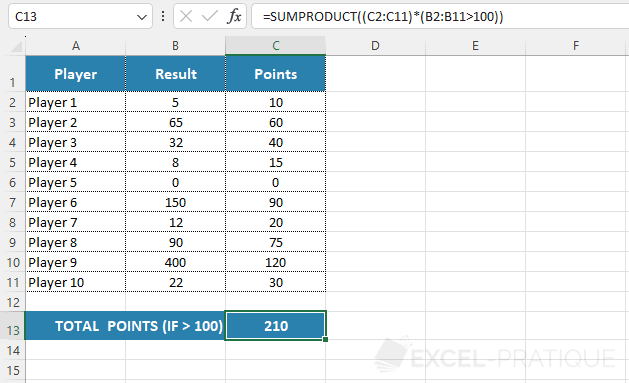To now sum the points whose result is `>100` or `<20`, the formula becomes:

``=SUMPRODUCT((C2:C11)*((B2:B11>100)+(B2:B11<20)))``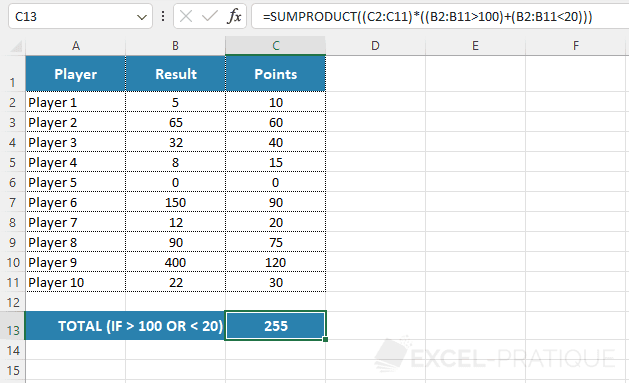To better understand `((B2:B11>100)+(B2:B11<20))`, remember that a test returns 0 or 1.

In this case, the ranges are added to obtain the range `((B2:B11>100)+(B2:B11<20))` which is the result of the addition of the 0s and 1s of the 2 ranges: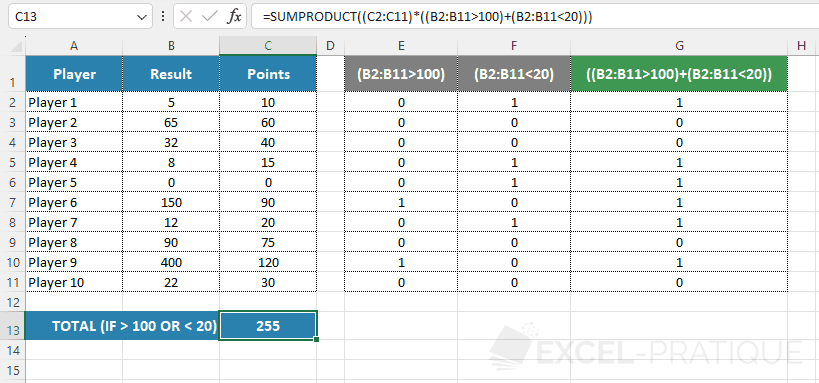If we now reverse the conditions to sum the points whose result is `<=100` and `>20`, it will not be enough to reverse the tests.

The following formula will not return the expected result:

``=SUMPRODUCT((C2:C11)*((B2:B11<=100)+(B2:B11>=20)))``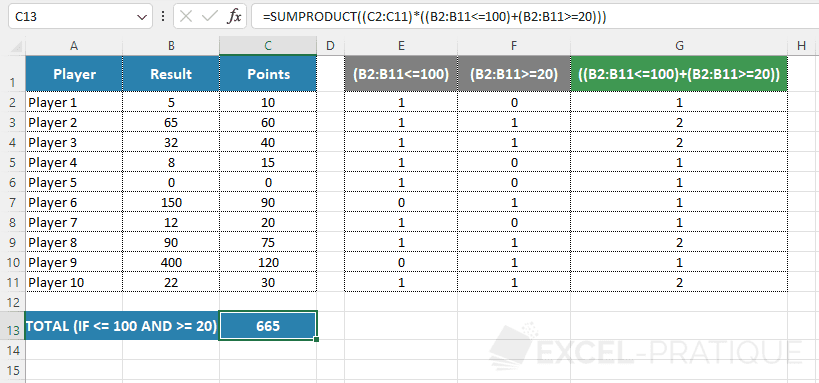For it to work, you need to add a condition to the range `((B2:B11<=100)+(B2:B11>=20))` to only keep those who have passed the 2 tests (thus those `=2`).

The formula therefore becomes:

``=SUMPRODUCT((C2:C11)*(((B2:B11<=100)+(B2:B11>=20))=2))``